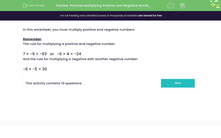# Practise Multiplying Positive and Negative Numbers

In this worksheet, students will practise multiplying positive and negative numbers.Key stage:  KS 3

Curriculum topic:   Number

Curriculum subtopic:   Use Four Operations for All Numbers

Difficulty level:#### Worksheet Overview

In this activity, we will be multiplying positive and negative numbers.

Remember

If you multiply one positive and one negative number, your answer will be negative.

If you multiply two negative numbers or two positive numbers, your answer will be positive.

Examples

-2 x 6 = -12

7 × -9 = -63

-6 × 4 = -24

-6 × -5 = 30

It's simple, if you remember those basic rules above!

Let's get started on some questions now.

### What is EdPlace?

We're your National Curriculum aligned online education content provider helping each child succeed in English, maths and science from year 1 to GCSE. With an EdPlace account you’ll be able to track and measure progress, helping each child achieve their best. We build confidence and attainment by personalising each child’s learning at a level that suits them.

Get started